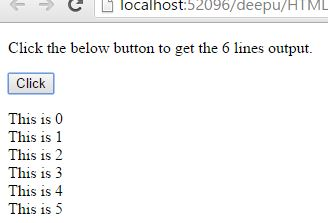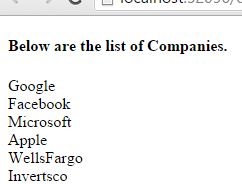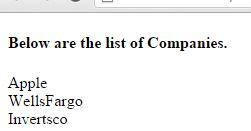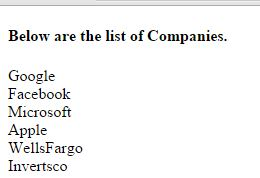# For loop in JavaScript

### For Loop

Loop is used to repeat a code block till a certain condition is true. If we want to run the same code again and again with different values we will use loops.

For loop is used to repeat a block of code a number of times with different values. For loop is having 3 parameters which are as follow.

• Parameter1 - The initial value to start.
• Parameter2 - Condition till the loop should execute.
• Parameter3 - Increment the value.

### Alerting 5 times continuosly by using For loop.

```   <script>
function TestFunction() {
var i = 5;
for (i = 0; i < 5; i++) {
}
}
</script>

<input type="button" id="btnTest" onclick="TestFunction()" value="Click me" />
```

In above code snippet, on click of button TestFunction() shall fire that will run a for loop. This loop executes five times and gives alert messages from 0 to 4. Click here to see the output of above code snippet.

In the output we can notice 5 alerts continuously one after the other.

### 6 lines outputs with the single code.

```<p>Click the below button to get the 6 lines output.</p>
<input type="button" value="Click" onclick="myLoops()" />
<p id="myId"></p>

<script>
function myLoops() {
var a = "";
var i;
for (i = 0; i < 6; i++){
a += "This is " + i + "<br/>";
}
document.getElementById("myId").innerHTML=a;
}
</script>
```

6 lines output

We need to return the 6 lines of output, for that we use `i<6` in For loop, Onclick of the myLoops() button gives 6 line of output.

OUTPUT### Inclucding parameter1 - The initial value to start.

In Foor loop Parameter1 (Initial value to start) is an optional parameter and it is used to initiate the variables used in the loop.

The above statement is not always the case, we can initiate many values in parameter1.

```<h4>Below are the list of Companies.</h4>
<p id="myId"></p>

<script>
var i, len, text;
for (i = 0, len = comp.length, text = ""; i < len; i++) {
text += comp[i] + "<br>"
}

document.getElementById("myId").innerHTML = text;
</script>
```

Returning the name of companies with parameter1

We are returning the companies names one after the other by including parameter1 (Giving i=0) in For loop.

OUTPUT### Omitting parameter1 (Values are set before the loop starts).

```<h4>Below are the list of Companies.</h4>
<p id="myId"></p>

<script>
var i = 3;
var len = comp.length;
var txt = "";

for (; i < len; i++) {
txt += comp[i] + "<br/>";
}

document.getElementById("myId").innerHTML = txt;
</script>  ```

Returning the name of the companies without parameter1

we are returning the companies names one after the other without including parameter1 (Given i=3)in For loop. So i=3 retruns from the 3rd company name. Numbering will go like this "Google=0, Facebook=1,.............Invertsco=5".

OUTPUT#### Prarameter2

Parameter2 is also an optional parameter in For loop, i.e condition till the loop should execute.

This will not same always. If the parameter2 returs true, the loop start from first, if the parameter2 returns false, the loop ends.

NOTE: If we want to omit the parameter2, we must declare the break inside the loop. Otherwise loop will never end, it leads to crash the browser.

#### Parameter3 - Increment the value

The 3rd parameter in For loop is increment the value and this parameter is also optional.

Increment the value is not always the case, it can do the any increment either negative (i--) or positive (i++) or etc. This parameter can also be omitted.

```<h4>Below are the list of Companies.</h4>
<p id="myId"></p>

<script>
var i = 0;
var len = comp.length;
var txt = "";

for (; i < len; i++) {
txt += comp[i] + "<br/>";
}

document.getElementById("myId").innerHTML = txt;
</script>
```

Increment the value

We are incrementing the returning companies value by giving value "0" to the i.

OUTPUTViews: 5088 | Post Order: 26